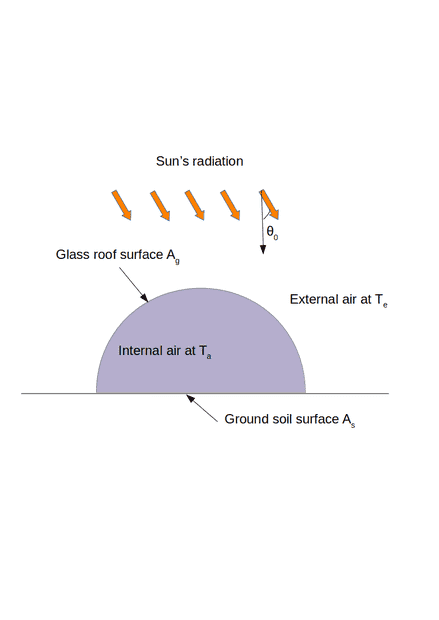# Average temperature in a greenhouse

• A
• Fefetltl

#### Fefetltl

TL;DR Summary
I am trying to compute the average temperature in a greenhouse taking into account solar radiation, internal and external thermal radiation and glass conduction loss
Hello everyone, since several weeks, no response from the other forums, I tried to compute a simple model for a greenhouse in a garden. First idea was to compute mass transfer, Navier-Stokes and heat equation all together but in my knowledge no analytical solution exists.

I need to build a simple model for an average temperature inside a greenhouse. That is why I consider only an average air temperature inside.

For this I use a 0D model and consider only a stationary power balance, neglecting natural convection loss (but I can add it to the model, just that this is not the blocking point):

$$P_{sun} + P_{radiation,in} - P_{radiation,out} - P_{conduction} = 0$$

According to the drawing, I consider only the 2 main surfaces of the greenhouse: soil surface ##A_s## and glass roof surface ##A_g##, for the 2 lateral wall I consider their surface negligible and their thermal conduction low to ignore conduction loss on these parts.

Let us decompose the powers:

## P_{sun} = \Phi_0 \cos \theta_0 A_s ##, scalar product between sun rays and ground soil surface (angle is zero at zenith)
##P_{radiation,in} \simeq \sigma T_a^4 ( \epsilon_g A_g + \epsilon_s A_s ) ##, approximated radiosity law for view factors
## P_{radiation,out} \simeq \sigma T_e^4 \epsilon_g A_g ##, because the glass roof loses energy to the external air
## P_{conduction} = \frac{\lambda_g A_g}{h_g} (T_a - T_e) ##, conduction loss between glass and exterior air, for simplicity we neglect conduction loss by the soil, because negligible compared to glass conduction loss

With these assumptions, thermodynamic first law (time derivative) gives at equilibrium:

$$\Phi_0 \cos \theta_0 A_s + \sigma T_a^4 ( \epsilon_g A_g + \epsilon_s A_s ) - \sigma T_e^4 \epsilon_g A_g - \frac{\lambda_g A_g}{h_g} (T_a - T_e) = 0$$

with ##h_g##, ##\lambda_g##, ##\epsilon_g## thickness, thermal conductivity and emissivity of glass. ##\epsilon_s##, ##T_a##, ##T_e##, ##\Phi_0##, ##\theta_0##: soil emissivity, inside air temperature, external air temperature, sun's power flux transmitted inside the greenhouse, angle between sun's rays and soil surface ##A_s##.

Because you can linearize the equation, i.e ##T_a^4 = (\tau_a + T_e)^4 \simeq T_e^4 + 4 \tau_a T_e^3## (with ## \tau_a << T_e ##), when you solve the first equation, and using that glass conduction is predominant we have:

$$T_a \simeq T_e + \frac{h_g}{\lambda_g A_g} \left( \sigma A_s T_e^4 + \phi_0 \cos(\theta_0) A_s \right)$$

The temperature found are pretty ok but the problem is as you can see that even without sun's radiation (##\Phi_0 = 0##): we still have ##T_a## different to ##T_e## at thermodynamics equilibrium. Maybe should I consider a non-stationary equation to take into account the radiation decay ? The internal infrared radiation should be zero at a moment, otherwise it is infinite energy inside the greenhouse, so there is a big problem with this stationary model... Or maybe my thermal flux are completely wrong ?

PS: I did also the same model at equilibrium using wall heat conduction loss, convection heat loss, with no approximation and that gives almost the same result
PPS: I did the same model for a greenhouse completely in glass and far away from the soil (stupid but just to verify the validity of the equation) and in this case, because there is no ground but just glass soil surface, the equilibrium is reached at ## T_a = T_e## when ##\phi_0 = 0##, so it seems good for this stupid imaginary case by the wayin the result.

Last edited:

Hello @Fefetltl ,##\qquad## !​

I can follow most of the reasoning. My suspicion is that your problem
we still have Ta different to Te at thermodynamics equilibrium
comes from having radiation coming in from the soil but not going out to the soil.
So ## \sigma T_a^4 ( \epsilon_s A_s ) ## is the term that forces ##\tau_a>0##.
If, as you say: the temperature found is pretty OK, perhaps you can accept that.

##\ ##

•Fefetltl
Hi Bvu and thank you for the welcome sign and your answer!

You catch the point right... that being said why not in fact? A perfect isolated greenhouse (no air leak), should experience a higher temperature inside because the soil does not radiate out as you just said. This taking the energy of the ground in such way... I dont know, finaly it does not chock me so much... thanks again to follow and replied.